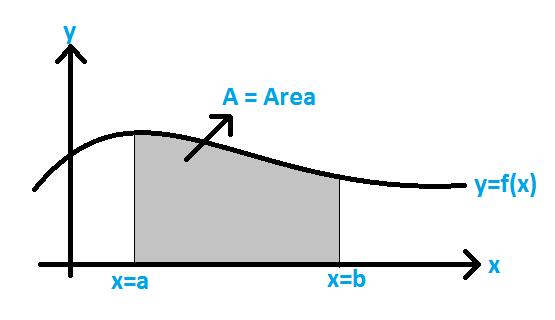# Area Under the Curve Formula

The area under a curve between two points is found out by doing a definite integral between the two points. To find the area under the curve y = f(x) between x = a & x = b, integrate y = f(x) between the limits of a and b. This area can be calculated using integration with given limits.## Formula to Calculate the Area Under a Curve

The formula for Area under the Curve  = ab f(x)dx

### Solved Example

Question : Calculate the area under the curve of a function, f(x) = 7 – x2, the limit is given as  x = -1 to 2.
Solution:

Given function is, f(x) = 7- x2 and limit is x = -1 to 2

$$\begin{array}{l}\large Area = \int_{-1}^{2}(7-x^{2})dx\end{array}$$

$$\begin{array}{l}\large = \left ( 7x-\frac{1}{3}x^{3}\right)|_{-1}^{2}\end{array}$$

$$\begin{array}{l}\large = \left [ 7.2-\frac{1}{3}(8) \right ]-\left [ 7(-1)-\frac{1}{3}(-1)\right ]\end{array}$$

= [(42 – 8)/3] – [(1 – 21)/3]

= (34 + 20)/3
= 54/3

= 18 sq.units